# himaginary's blog

## 2009年4月25日土曜日

### What happened in Japan in 2003 ?

Below is citation from Krugman's article 10 years ago.

A rigorous model of monetary policy in the face of imperfect substitution is difficult to construct (if only because one must derive that imperfection somehow). But a shortcut may be useful. Consider, then, the case of foreign exchange intervention – purchasing foreign bonds in an effort to bid down the currency. And let us look back at Figure 5, which illustrates how a liquidity trap can occur even in an open economy, because the desired capital export even at a zero interest rate will be less than the excess of domestic savings over investment.

What would the central bank be doing if it engages in exchange-market intervention in such a situation? The answer is that in effect it would be trying to do through its own operations the capital export that the private sector is unwilling to do. So a minimum estimate of the size of intervention needed per year is "enough to close the gap" – that is, the central bank would have to buy enough foreign exchange , i.e. export enough capital, to close the ex ante gap between S-I and NX at a zero interest rate. In practice, the intervention would have to be substantially larger than this, probably several times as large, because the intervention would induce private flows in the opposite direction. (An intervention that weakens the yen reinforces the incentive for private investors to bet on its future appreciation).

Here is some sample arithmetic: suppose that you believe that Japan currently has an output gap of 10 percent, which might be the result of an ex ante savings surplus of 4 or 5 percent of GDP. Then intervention in the foreign exchange market sufficient to close that gap would have to be several times as large as the savings surplus – i.e., it could involve the Bank of Japan acquiring foreign assets at the rate of 10, 15 or more percent of GDP, over an extended period. (Incidentally, does it matter if the interventions are unsterilized? Well, an unsterilized intervention is a sterilized intervention plus quantitative easing; the latter part makes no difference unless it affects expectations).

Many people think that, in 2003, Japan actually did what Krugman wrote in the above article: i.e. MOF (Ministry of Finance) did huge sum of exchange-market intervention, bid down the currency, and exported enough capital to close the ex ante gap between S-I and NX at a zero interest rate.

Did it really happen? Let's check the data.

Below is the graph of international account. In 2003, huge sum of exchange-market intervention did take place -- although did not exceed 10% of GDP as Krugman suggested, but still reached about 7% of GDP, 34 trillion yen. However, it was only one-year intervention, and was not conducted over an extended period as Krugman suggested.Did it bid down the currency?
Below is the monthly data of Change in Forex Reserves and JPY/USD exchange rate from 2002 to 2004. You can see that it was far from bidding down the currency -- if anything, all it did was dampen the speed of currency appreciation, at most.And how did it effect the monetary base?
If you see the below graph carefully, although monetary base increased during 2002-2004, you can see that the increase was not necessarily due to forex intervention. All-out intervention began from mid-2003, but a large part of the increase in monetary base was already made by then.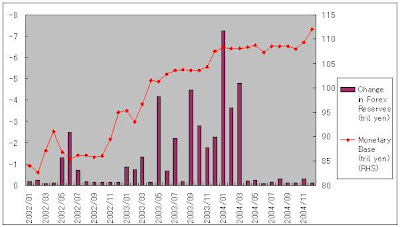In sum, the gigantic forex intervention in 2003 did not bid down the currency, and it did not lead to quantitative easing by itself. Then what did it accomplish? Did it close the ex ante gap between S-I and NX?

Maybe. In fact, current surplus increased in 2003. However, the most striking effect of 2003 was that capital account ran large surplus. That is, the intervention caused import of capital, not export of capital as Krugman predicted.

What Krugman suggested as the forex intervention effect was closing the gap between S-I and NX by increasing NX. NX did increase a little, but the most part of the intervetion was consumed by change in capital account, which transformed from deficit to large surplus in 2003. The capital surplus meant that the foreigners invested in Japan, which turned into the increase in I. So if ex ante gap between S-I and NX was closed in Japan, it was caused not by increase in NX as Krugman suggested, but by increase in I!

In other words, the government financed the foreign investment in Japan, which lead to economic recovery. It was not what Krugman predicted. Krugman's theory was that the government finances export, which brings about export-led recovery.

In fact, if you look at the GDP data, you can see that investment was important factor of economic growth on and after 2003. Yes, net export also seems to have played a role, but actually it didn't. I will treat that matter in another post.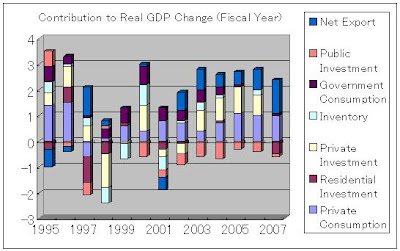## 2009年4月20日月曜日

### Weight P/E by P*E ?

A while ago, Jeremy Siegel attacked P/E ratio calculated by S&P for overestimating the real value (here and here. HT Greg mankiw's Blog).

S&P are now using the following formula: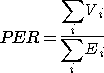where Vi is the market value and Ei is the earning of issue i, and PER denotes P/E ratio.
Siegel asserts that S&P should weight both market value(numerator) and earning(divisor) by market value, such as:Siegel says that this weighting will alleviate the problem of overestimating the overall P/E ratio, because the huge loss of the company like AIG will be downplayed by its relatively small market value, in comparison with S&P formula .

However, when you transform S&P formula as follows, you can see that it is also a kind of weighted sum:That is, individual P/E ratio is weighted by earning.

When you look at Seigel's formula, it can be re-written as follows: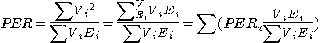That is, individual P/E ratio is weighted by the product of value and earning.

It can also transformed as follows: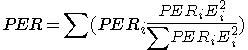Individual P/E ratio is weighted by the product of P/E and squared earning.

From these transformations, you can see that the meaning of Siegel's formula is much harder to grasp than S&P one.

For Siegel's purpose, maybe it's better to weight individual earning by market value, instead of by earning in S&P formula, as follows:## 2009年4月12日日曜日

### The Geithner put : simulation

In the previous post, I showed the way to simulate Geithner plan by Excel for three probability density functions(uniform, normal, log-normal). In this post, I show some graphs from the simulation.

Here I use log-normal PDF, for it is the most probable function of the three.
Specifically, I assumed that ln(P) follows N[m,s] distribution, and set mean m as ln(100)-s2/2. That means expected value of P (=E[P]) is always set as 100.

• standard deviation simulation

First, change standard deviation s from 0.1 to 1.1 by 0.05. Self financing ratio k is set as 1/7 as in the Geithner plan.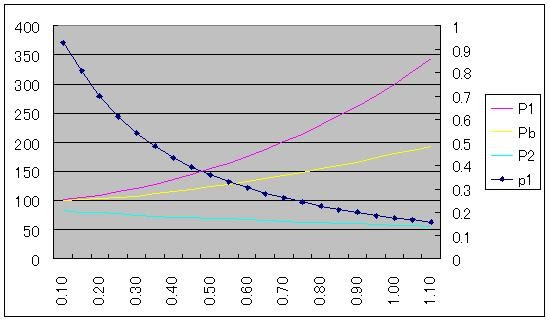X-axis is the simulation factor s, as described above.
P1 is the expected price conditional that non-recourse loan not be used.
P2 is the expected price conditional that non-recourse loan be used.
Pb is the break-even bid price.
p1(right-hand scale) is the probabaility that non-recourse loan not be used.

As s increases, P1 and Pb goes up, and P2 decreases gradually.
p1 decrease from over 0.9 to near 0.1 for the simulated range of s.

The graph below shows taxpayer's loss (FDIC's loss, to be exact) as percentage of (1-k)Pb(=the value of FIDC non-recourse loan), instead of p1 for the same simulation (right-hand scale). X-axis is same as above, but numbers are changed from s to s/m (coefficient of variance) so that the meaning of x-axis becomes more easy to grasp.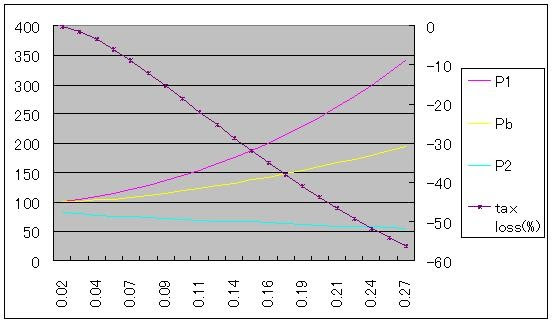For coefficient of variance of 0.27, FDIC's loss ratio reaches near 60%.

• non-recourse loan ratio simulation

Next, change self financing ratio k(=1 - non-recourse loan ratio) from 0.05 to 0.95 by 0.05 (this includes Treasury funding). s(standard deviation) is set as 0.5.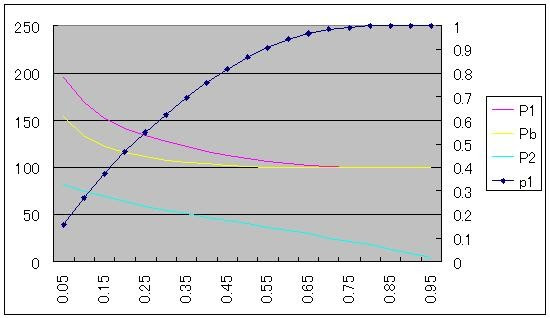(Again, p1 is right-hand scale.)

When k increases from 0.15 to 0.45, Pb premium to E[P] decreases from 22.0% to 2.3%. Maybe low k value(1/7=14.3%) in the Geithner plan was set in order to provide enough (over 20%) subsidy to the bank.

FDIC's loss ratio is as follows.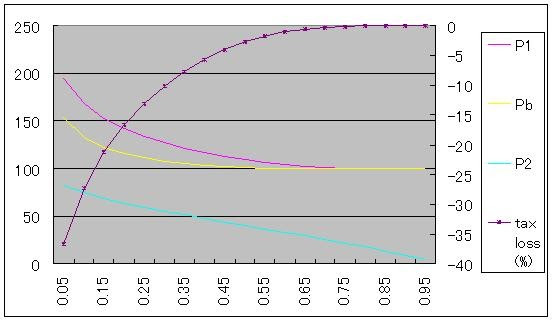(Again, loss ratio is right-hand scale.)

If non-recourse loan ratio (1-k) is about 75% instead of 85.7% in the Geithner plan, the loss drops in half (around 20% to 10%). If (1-k) is 50%, loss becomes less than 3%.

## 2009年4月11日土曜日

### The Geithner put : general PDF case

In the previous two posts, I showed general formula of break-even purchase price and fund's gain/loss under the Geithner plan. I also did some arithmetic using two-state model.

Krugman wrote "the natural way to think about these things is in fact in terms of simple two-state numerical examples." Nemo commented "binomial distribution is oversimplified."

However, two-state model may be not so simple as it seems. It can be described as reduced form of more general case.

Let p(P) the probability density function(PDF) of P. Then P1, P2, p1 in the two-state model I used can be derived from p(P) as follows: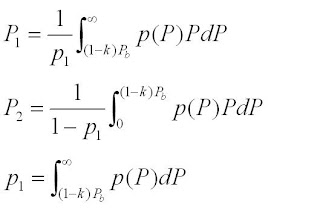where Pb is break-even purchase price, and (1-k) is ratio of non-recourse loan offered by FDIC.

As derived here, Pb is function of P1 and p1. That is, Pb = p1P1 / (p1+k(1-p1)). So Pb and P1, p1 must be calculated simultaneously.

As for general PDF, that calculation can be done by Excel. Below I show how to do the calculation by Excel for three forms of p(P), i.e. uniform distribution, normal distribution, log-normal distribution.

• uniform distribution

p1P1Pb
Excel row/columnABC
2Initial valueInitial value=A2*B2/(A2+\$G\$2*(1-A2))
3=(\$I\$2-C2*(1-\$G\$2))/(\$I\$2-\$H\$2)=(\$I\$2^2-(C2*(1-\$G\$2))^2)/2/(\$I\$2-\$H\$2)/A3=A3*B3/(A3+\$G\$2*(1-A3))

Input k in G2 cell, and upper limit of uniform distribution in I2 cell, lower limit in H2 cell.
If you drag fill handle of 3rd row to appropriate row (say, 41st row), then each value of Pb, P1, p1 will converge.

• normal distribution

p1P1Pb
Excel row/columnABC
2Initial valueInitial value=A2*B2/(A2+\$G\$2*(1-A2))
3=1-NORMDIST(C2*(1-\$G\$2),\$H\$2,\$I\$2,TRUE)=(EXP(-((((1-\$G\$2)*C2-\$H\$2)/\$I\$2))^2/2)*\$I\$2/SQRT(2*PI())+\$H\$2*A3)/A3=A3*B3/(A3+\$G\$2*(1-A3))

Input k in G2 cell, and mean of normal distribution in H2 cell, standard deviation in I2 cell.
If you drag fill handle of 3rd row to appropriate row (say, 41st row), then each value of Pb, P1, p1 will converge (if they ever do).

(Note: Above Excel formula is based on normal distribution without restriction, so price could be negative.)

• log-normal distribution

p1P1Pb
Excel row/columnABC
2Initial valueInitial value=A2*B2/(A2+\$G\$2*(1-A2))
3=1-LOGNORMDIST(C2*(1-\$G\$2),\$H\$2,\$I\$2)=(1-NORMDIST( (LN(C2*(1-\$G\$2))-\$H\$2)/\$I\$2,\$I\$2,1,TRUE))*EXP(\$I\$2^2/2+\$H\$2)/A3=A3*B3/(A3+\$G\$2*(1-A3))

Input k in G2 cell, and mean of log-normal distribution in H2 cell, standard deviation in I2 cell.
If you drag fill handle of 3rd row to appropriate row (say, 41st row), then each value of Pb, P1, p1 will converge (if they ever do).

### The Geithner put : option formula

In the previous post , I showed the general formula of break-even purchase price under Geithner plan.

In that post, I showed the gain/loss of the investment fund as follows:
w(P - Pb)　　　if P >= (1-k)Pb
-wPb　　　　　 if P < (1-k)Pb
where:
P is true price of the loan,
Pb is the purchase price,
(1-k) is ratio of non-recourse loan offered by FDIC (6/7 in case of Geithner plan),
w is ratio of fund money in investment (1/2 in case of Geithner plan; the rest(=1-w) is funded by Treasury).

It also can expressed in option formula as follows:
w{Max(P-Pb , -kPb)}
=w{Max(P-(1-k)Pb , 0)-kPb}

This is the formula of call option with strike price=-(1-k)Pb and option purchase price=kPb (See image below).By applying the above option formula, it can be calculates as
0.5*{(0.5*(100-84*6/7)+0.5*0)-84/7}=1
which corresponds to Nemos's result.

As Nemo showed, the money fund put in is wkPb=0.5*1/7*84=6. So the fund performance reaches 1/6*100=16.67%.

FDIC gain/loss also can be expressed in option formula as follows:
-Max(0 , (1-k)Pb-P)
(In fact, maximum gain is not zero, as there is interest revenue. Here I ignore it for simplicity)

This is the formula of put option with strike price=-(1-k)Pb (See image below).The FDIC position is short on this put option, and that "Geithner put" is what enables the floor part of the fund's call option shown above.

## 2009年4月10日金曜日

### The Geithner put : general formula

Many people have been calculating the effect of Geithner put in the Geithner plan (aka PPIP) . However, while many have shown numerical examples, seldom of them have shown the general formula. So I am going to give one.

Usually, when a fund purchases loan from bank, its gain/loss can be written as
P - Pb
where P is true price of the loan, and Pb is the purchase price.
Therefore, expected return of the fund becomes zero when Pb=E[P]
(E[.] denotes expectation value).

But with Geithner put, the gain/loss of the fund becomes
w(P - Pb)　　　if P >= (1-k)Pb
-wPb　　　　　 if P < (1-k)Pb
where:
(1-k) is ratio of non-recourse loan offered by FDIC (6/7 in case of Geithner plan),
w is ratio of fund money in investment (1/2 in case of Geithner plan; the rest(=1-w) is funded by Treasury).

In this case, Pb, the break-even purchase price, is not necessarily E[P].

Now let P becomes P1 with probability p1, and P2 with probability 1-p1.
(Assumption: P1 >= (1-k)Pb > P2)

Then the break-even purchase price can be written as
Pb = p1P1 / (p1+k(1-p1))

RHS does not include P2, which means one does not have to consider P2 as for down-side risk. This is because if down-side event really happens, the price P2 does not affect the loss of the fund; the fund just loses the money it put in.

Let confirm this formula by Krugman's example. In Krugman's case, p1=0.5, P1=150, k=0.15, so Pb becomes 130.43, which corresponds to Krugman's calculation.

Jeffrey Sachs also showed numerical example. His setting is p1=0.2, P1=1,000,000, k=0.1. Then Pb becomes 714,268, which corresponds to Sachs's calculation.

And in this case, break-even price Pb is always higher than expectation value of P. That result can be shown by the following arithmetic:

Pb-E[P]
=p1P1 / (p1+k(1-p1)) - (p1P1+(1-p1)P2)
=p1P1 [1/{p1+k(1-p1)} -1] - (1-p1)P2
=p1P1[(1-k)(1-p1)/{p1+k(1-p1)}] - (1-p1)P2
=(1-p1)[p1P1(1-k)/{p1+k(1-p1)} - P2]
=(1-p1)[(1-k)Pb-P2]

This value is always positive by assumption. And this value represents the "subsidy" to the bank under the Geithner plan.

And if the purchase price is higher than break-even price Pb by, say, R, it adds to that subsidy to the bank.
In that case, the private invester loss is -wR(p1+(1-p1)k), Treasury loss is -(1-w)R(p1+(1-p1)k).
And FDIC loss becomes larger by -R(1-(p1+(1-p1)k)).
Graph below shows the loss of the three stake holders as function of p1 (total=100%; k=1/7, a=1/2 as in the Geithner plan).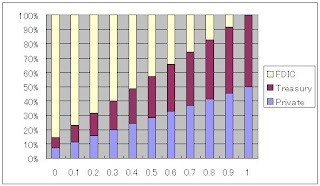Loss of FDIC+Treasury makes up 80% of total when p1=0.3, and becomes less than 70% only when p1 is larger than 0.6.

## 自己紹介

This blog is some thoughts on economics by a Japanese non-economist. Translated from my Japanese blog.